# Locally algebraic operator

(diff) ← Older revision | Latest revision (diff) | Newer revision → (diff)

A linear operator such that for each element of the space under consideration there exists a polynomial in this operator (with scalar coefficients) annihilating this element.

Letbe a linear space over a field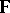. Let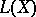be the set of all linear operators with domains and ranges inand let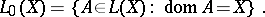Denote by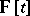the algebra of all polynomials in the variableand with coefficients in. Usually, in applications it is assumed that the fieldis of characteristic zero and algebraically closed (cf. also Algebraically closed field; Characteristic of a field).

Thus, a linear operator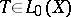is said to be locally algebraic if for anythere exists a non-zero polynomialsuch that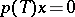(cf. [a1]). If there exists a non-zero polynomial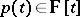such thatfor every, thenis said to be algebraic (cf. Algebraic operator). Thus, an algebraic operator is locally algebraic, but not conversely.

A continuous locally algebraic operator acting in a complete linear metric spaceis algebraic (cf. [a4]; for Banach spaces, see [a1]).

A locally algebraic operatoracting in a complete linear metric space(over the fieldof complex numbers) and that is right invertible (cf. Algebraic analysis) but not invertible, i.e.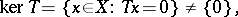is not continuous (cf. [a3]). The assumption about the completeness ofis essential.

Ifsatisfies, for any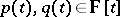, the conditions:

i)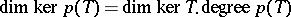;

ii);

iii)is locally algebraic; then there exists an operator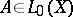such that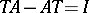(cf. [a2], [a5]). This means thatis not an algebraic operator.

How to Cite This Entry:
Locally algebraic operator. Encyclopedia of Mathematics. URL: http://encyclopediaofmath.org/index.php?title=Locally_algebraic_operator&oldid=13689
This article was adapted from an original article by Danuta Przeworska-Rolewicz (originator), which appeared in Encyclopedia of Mathematics - ISBN 1402006098. See original article